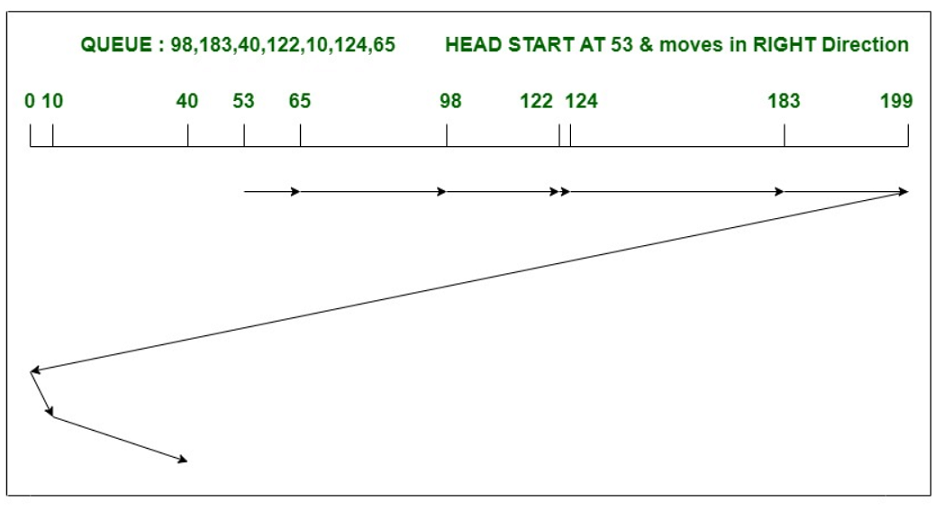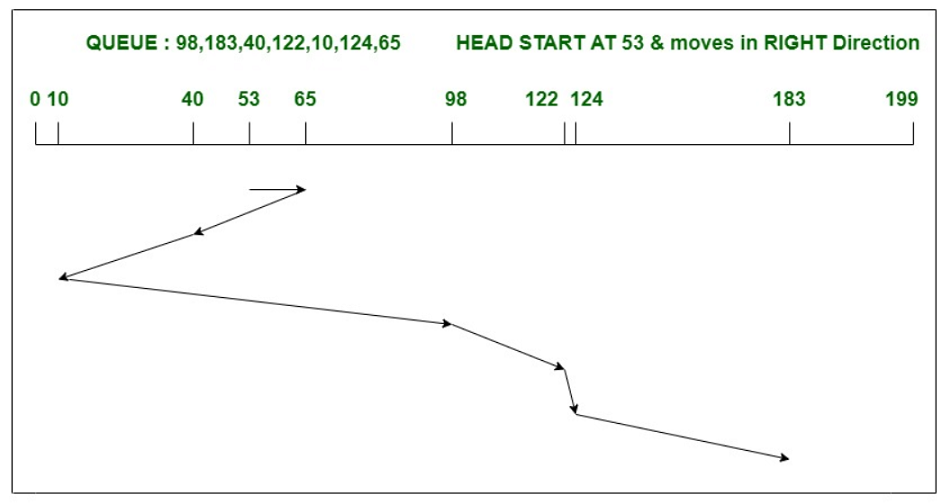# Difference between C-SCAN and SSTF Disk Scheduling Algorithm

• Last Updated : 01 Jul, 2022

1. C-SCAN Disk Scheduling Algorithm : C-SCAN algorithm, also known as Circular Elevator algorithm is the modified version of SCAN algorithm. In this algorithm, the head pointer starts from one end of disk and moves towards the other end, serving all requests in between. After reaching the other end, the head reverse its direction and go to the starting point. It then satisfies the remaining requests, in same direction as before. Unlike SSTF, it can handle requests only in one direction.

Example – Consider a disk with 200 tracks (0-199) and the disk queue having I/O requests in the following order as follows:

`98, 183, 40, 122, 10, 124, 65 `

Current head position of the Read/Write head is 53 and will move in Right direction . Calculate the total number of track movements of Read/Write head using C-SCAN algorithm.Total head movements,

```= (65-53)+(98-65)+(122-98)+(124-122)+(183-124)
+(199-183)+(199-0)+(10-0)+(40-10)
= 395 ```

2. SSTF Disk Scheduling Algorithm : SSTF stands for Shortest Seek Time First, as the name suggests it serves the request which is closest to the current position of head or pointer. In this algorithm, direction of  the head pointer matters a lot. If there occurs a tie between requests, then the head will serve the request in its ongoing direction. Unlike C-SCAN, SSTF algorithm is very efficient in total seek time.

Example – Consider a disk with 200 tracks (0-199) and the disk queue having I/O requests in the following order as follows:

`98, 183, 40, 122, 10, 124, 65 `

Current head position of the Read/Write head is 53 and will move in Right direction. Calculate the total number of track movements of Read/Write head using SSTF algorithm.Total head movements,

```= (65-53)+(65-40)+(40-10)
+(98-10)+(122-98)+(124-122)+(183-124)
= 240 ```

Difference between C-SCAN and SSTF Disk Scheduling Algorithm :

My Personal Notes arrow_drop_up